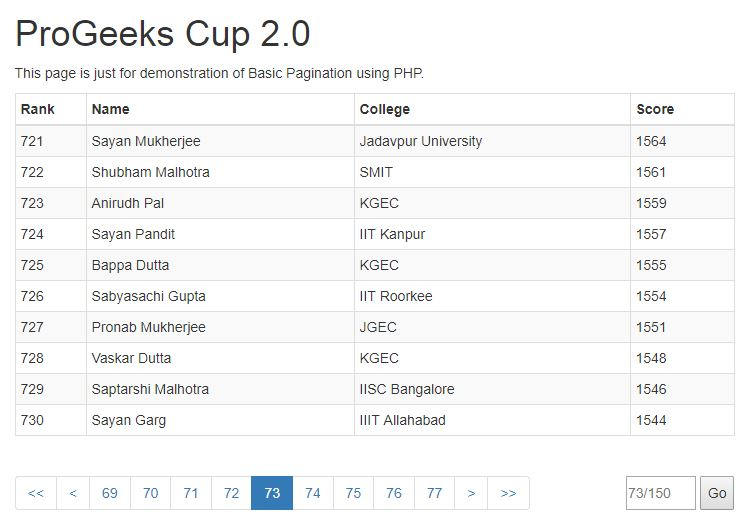# PHP Pagination | Set 3

• Difficulty Level : Medium
• Last Updated : 22 Mar, 2018

In the previous article PHP Pagination | Set 2, we developed a simple pagination system that was logically correct but it was not visually appealing because of all the page numbers stacked and occupied a lot of screen space. Then what is the remedy? It is simple when a user is looking up a particular page number it can be assumed that he will be moving in a sequential way. For example, if you are on page number 12 we can assume that you will go nearby pages not directly to page number 122. Assuming this, many pagination systems are evolved and made visually appealing as well. Let us take GeeksforGeeks Pagination system into account. The following figure is of the same.As you can see, the GeeksforGeeks Pagination system is a lot better it has an option to see the total number of pages. It has a last and next button as well, and similarly previous and the first button is also present dynamically i.e. based on current page number the combination of these buttons are shown. Now, before beginning to enhance our own pagination system we should note down what are the features that we should go for.

Analysis of Features

• First and foremost, we don’t want to show 150 pages, we will only show a local set of pages based upon the current page number.
• We should also have a previous/next and first/last button for quick navigation.
• If you think, a user’s movements can be classified into three classes. Sequential, Random, and Hybrid. By creating a link to local pages and the next/previous button we have taken care of the Sequential Movement. For Random movement, we should have an input field where the user may type in the particular page number and the rest of the navigation links should change accordingly.

As we have identified the features to be added, let us jump to the final source code and explain how we achieved the requirements.

Final Source Code

 `````  ````    ``ProGeeks Cup 2.0``    ````    ````    ````    ````  ````  ````  ````  ``
``    ``
``    ``
``      ``

ProGeeks Cup 2.0

``      ``

This page is just ``for` `demonstration of Basic Pagination using PHP.

``      ````        ``
RankNameCollegeScore
``        ````          ````          ````          ````          ````        ````        ````        ``
``        ``  ``        ``  ``          ``  ``          ````          ````          ``                                        ``        ``  ``        ``  ``        ````      ````      ``
``      ``
``      ````\$total_pages``)?``\$total_pages``-4:((``\$pn``-4<1)?5:``\$pn``));        ``        ``\$pagLink` `= ``""``;``        ``if``(``\$pn``>=2){``            ``echo` `"
• <<
• "``;``            ``echo` `"
• <
• "``;``        ``}``        ``for` `(``\$i``=-4; ``\$i``<=4; ``\$i``++) {``          ``if``(``\$k``+``\$i``==``\$pn``)``            ``\$pagLink` `.= ``"
• "``.(``\$k``+``\$i``).``"
• "``;``          ``else``            ``\$pagLink` `.= ``"
• "``.(``\$k``+``\$i``).``"
• "``;  ``        ``};  ``        ``echo` `\$pagLink``;``        ``if``(``\$pn``<``\$total_pages``){``            ``echo` `"
• >
• "``;``            ``echo` `"
• >>
• "``;``        ``}    ``      ``?>``      ``
``      ``
``      ``"` `      ``placeholder=``""` `required>``      ````      ``
``     ``
``    ````  ````  ````  `````

Whoa! That is too big to understand! Fear not, we have only included those that are highlighted and that also includes some CSS styling, some new HTML markup and a bit of logic. Let us not wait any further in explaining what we have changed and unveil the final result.

Code for Sequential Movement

The idea behind this code is very simple. We want to take a middle index \$k and we want to print the neighbor pages to create the sequential links. Before echoing the sequence we echo the previous and first-page links if the current page is not the first page. Similarly, after echoing the sequence we echo the next and last-page links if the current page is not the last page. In this case, we intend to show a total of 9 links of pages that form the sequence.

 `// K is assumed to be the middle index.``\$k` `= ((``\$pn``+4>``\$total_pages``)?``\$total_pages``-4:((``\$pn``-4<1)?5:``\$pn``));     `` ` `// Show prev and first-page links.``if``(``\$pn``>=2){``  ``echo` `"
• <<
• "``;``  ``echo` `"
• <
• "``;``}`` ` `// Show sequential links.``for` `(``\$i``=-4; ``\$i``<=4; ``\$i``++) {``  ``if``(``\$k``+``\$i``==``\$pn``)``    ``\$pagLink` `.= ``"
• "``.(``\$k``+``\$i``).``"
• "``;``  ``else``    ``\$pagLink` `.= ``"
• "``.(``\$k``+``\$i``).``"
• "``;  ``};  ``echo` `\$pagLink``;`` ` `// Show next and last-page links.``if``(``\$pn``<``\$total_pages``){``  ``echo` `"
• >
• "``;``  ``echo` `"
• >>
• "``;``}`

Code for Random Movement

The idea behind this is also fairly simple, we will provide an input field where the user can input any numerical value, after which we will check if the given value is valid and then we will go to the page. This doesn’t require much of PHP as it can be done using JS. PHP is used only to give us the value of \$total_pages present etc. In order to develop the above-mentioned feature, we first added the following markup and then added the function to handle click event.

 `<``div` `class``=``"inline"``>``  ``<``input` `id``=``"pn"` `type``=``"number"` `min``=``"1"` `max=""``         ``placeholder="" required>``  ``<``button` `onclick``=``"go2Page();"``>Go```
 `function` `go2Page()``{``    ``var` `pn = document.getElementById(``"pn"``).value;``    ``// Check if pn is between the max and min.``  ``pn = ((pn>)?:((pn<1)?1:pn));``  ``window.location.href = ``'index.php?page='` `+ pn;``}`

The rest of the updates are just some CSS to make it look a bit regular. Lastly, the final result that we get is shown in the figure below.I had a blast creating this Pagination system and am quite happy that it turned out to be decent. In case you need the source files and dataset, feel free to visit my repository. Happy Learning.

My Personal Notes arrow_drop_up